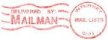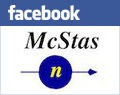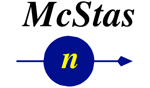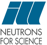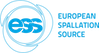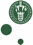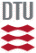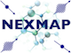McStas: Guide_channeled Component

# The `Guide_channeled` Component

Neutron guide with channels (bender section).

## Identification

• Author: Christian Nielsen
• Origin: Risoe
• Date: 1999
• Version:(Unknown)

## Description

```Models a rectangular guide tube centered on the Z axis. The entrance lies
in the X-Y plane.
The guide may be tapered, and may have vertical subdivisions (used for
bender devices).

There is a special rotating mode in order to approximate a Fermi Chopper
behaviour, in the case the neutron trajectory is nearly linear inside the
chopper slits, i.e. the neutrons are fast w/r/ to the chopper speed.
Slits are straight, but may be super-mirror coated. In this case, the
component is NOT centered, but located at its entry window. It should then
be shifted by -l/2.

Example: Guide_channeled(w1=0.1, h1=0.1, w2=0.1, h2=0.1, l=2.0,
R0=0.99, Qcx=0.0219, Qcy=0.0219, alphax=6.07, alphay=6.07, W=0.003, nslit=1,
d=0.0005, mx=1, my=1)

%BUGS
This component does not work with gravitation on. Use Guide_gravity.
This component does not work in multichannel focusing geometry.

Example values: mx=4 my=2 Qcx=Qcy=0.0219 W=1/300 alphax=alphay=6.49 R0=1

```

## Input parameters

Parameters in boldface are required; the others are optional.
Name Unit Description Default
w1 m Width at the guide entry
h1 m Height at the guide entry
w2 m Width at the guide exit 0
h2 m Height at the guide exit 0
l m Length of guide
R0 1 Low-angle reflectivity 0.995
Qc AA-1 Critical scattering vector 0
alpha AA Slope of reflectivity 0
m 1 m-value of material. Zero means completely absorbing. 0
nslit >= 1  Number of channels in the guide 1
d m Thickness of subdividing absorbing walls 0.0005
Qcx AA-1 Critical scattering vector for left and right vertical mirrors in each channel 0.0218
Qcy AA-1 Critical scattering vector for top and bottom mirrors 0.0218
alphax AA Slope of reflectivity for left and right vertical mirrors in each channel 4.38
alphay AA Slope of reflectivity for top and bottom mirrors 4.38
W AA-1 Width of supermirror cut-off for all mirrors 0.003
mx 1 m-value of material for left and right vertical mirrors in each channel. Zero means completely absorbing. 1
my 1 m-value of material for top and bottom mirrors. Zero means completely absorbing. 1
nu Hz Rotation frequency (round/s) for Fermi Chopper approximation 0
phase deg Phase shift for the Fermi Chopper approximation 0

## Output parameters

Name Unit Description Default
w1c
w2c
ww
hh
whalf
hhalf
lwhalf
lhhalf